Calculus II

Day 18-Lecture 36

6 April 2017, Thursday 9:40

Previous Screenshot       Next Screenshot

Change of variables in multiple integrals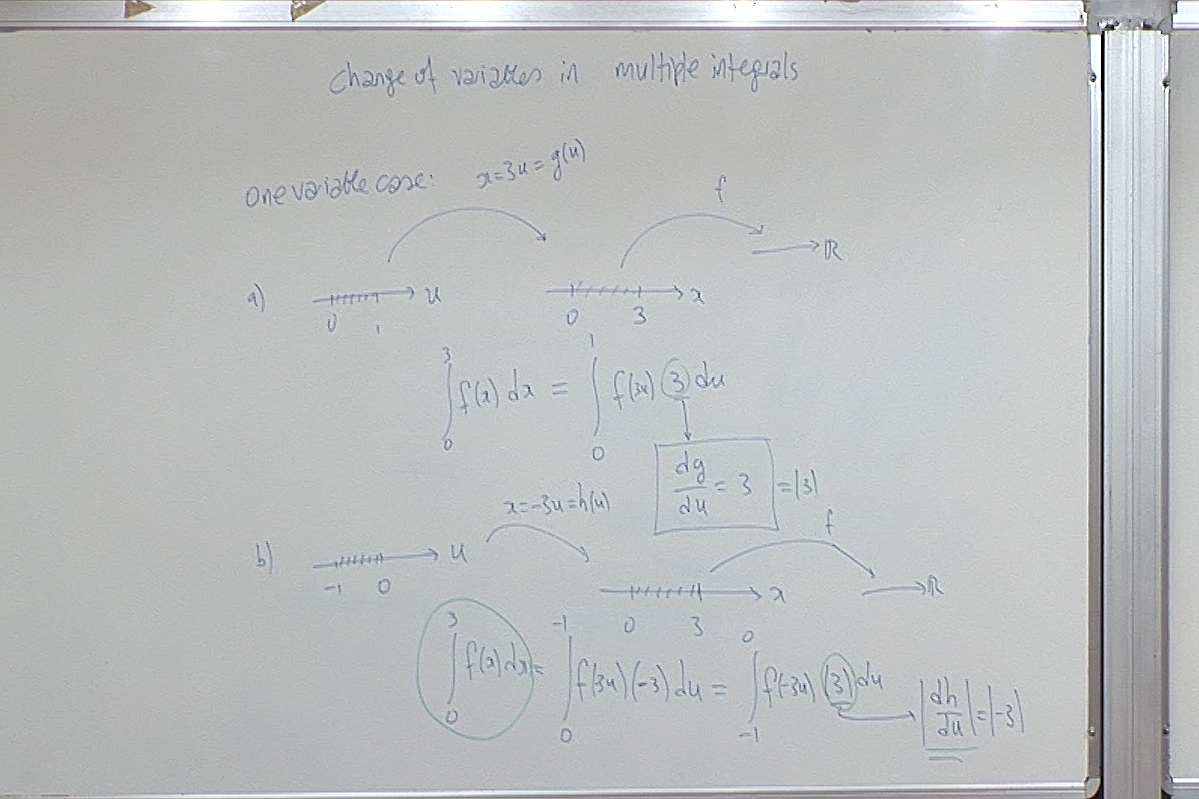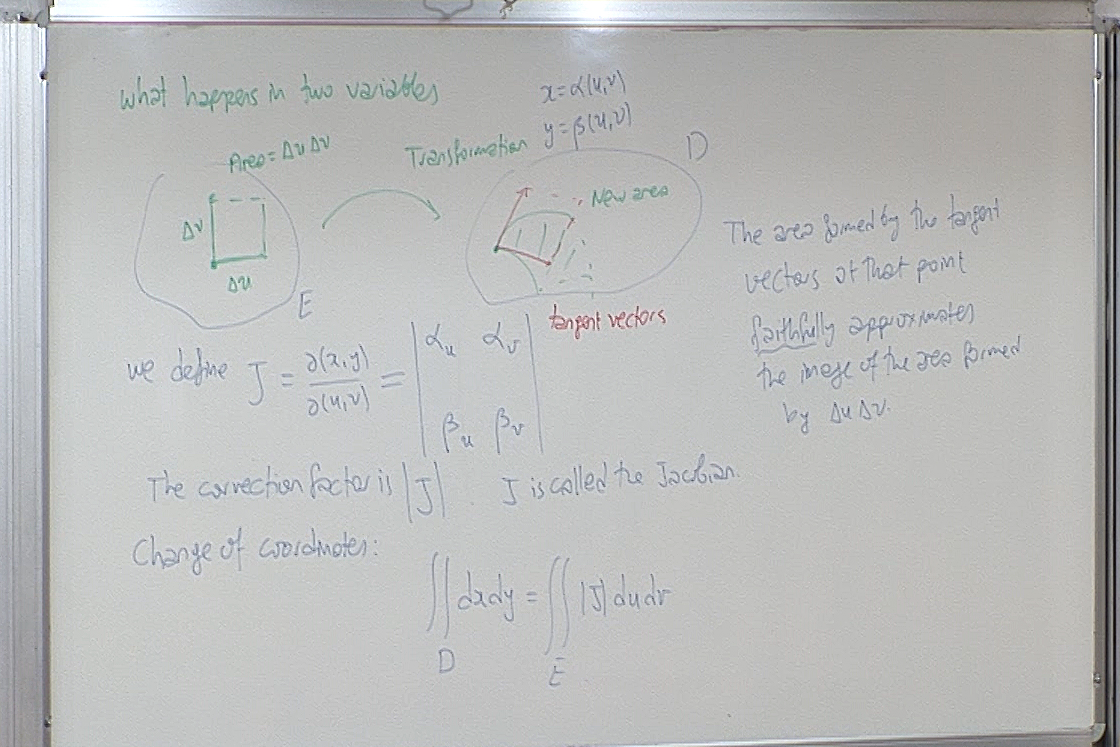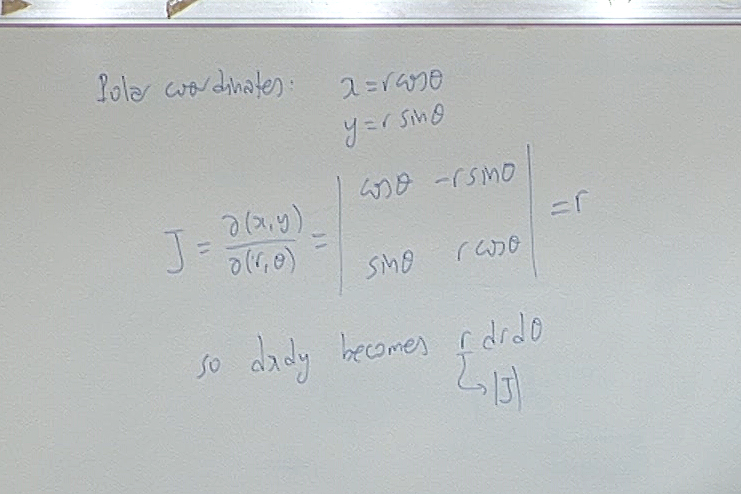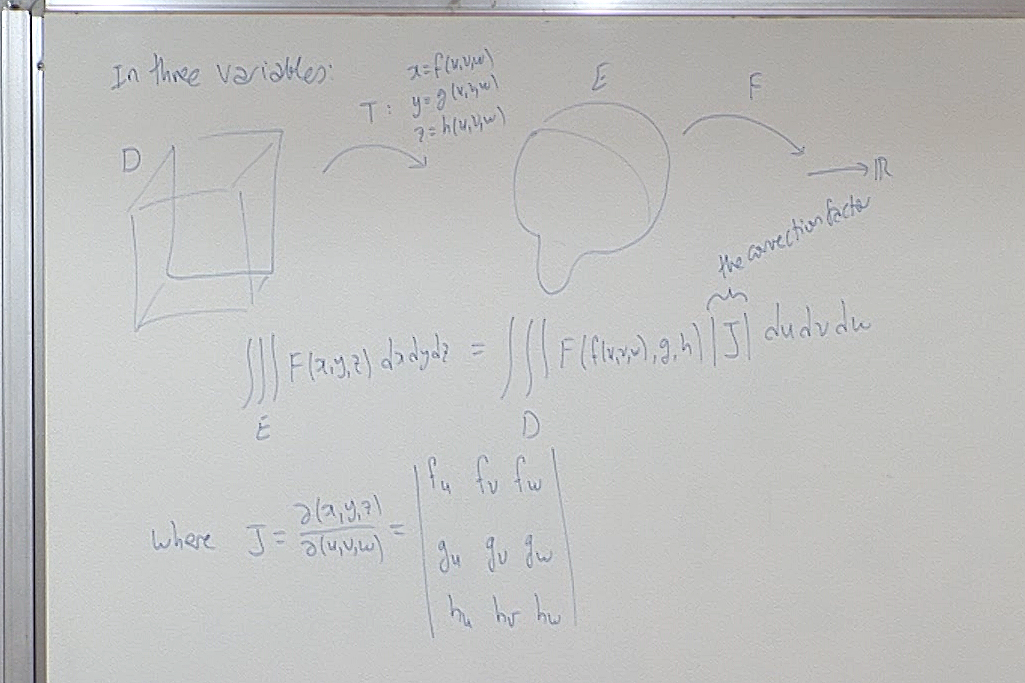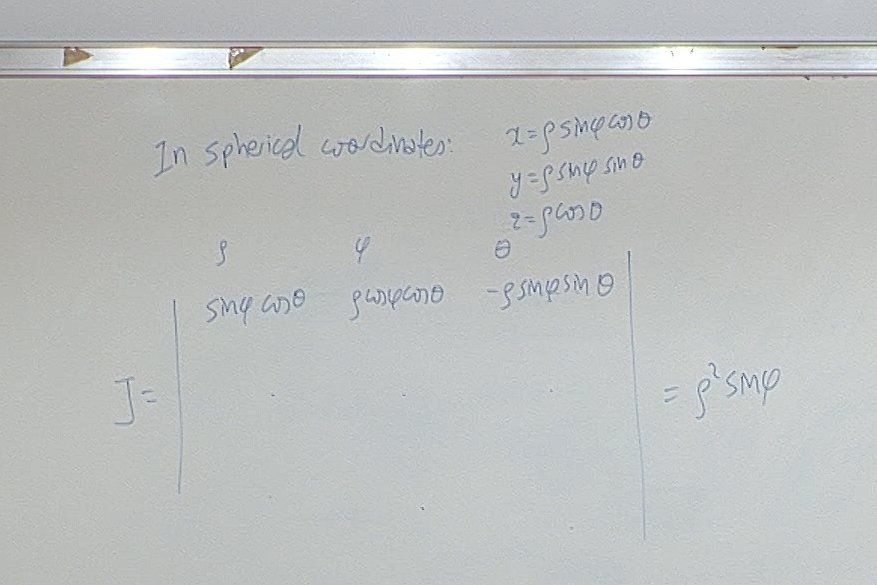When you complete the empty cells of the above Jacobian you will get $\begin{vmatrix} \sin\phi \cos\theta & \rho\cos\phi \cos\theta &-\rho\sin\phi \sin\theta \\ \sin\phi\sin\theta & \rho\cos\phi\sin\theta & \rho\sin\phi\cos\theta \\ \cos\theta &0 & -\rho\sin\theta \end{vmatrix} =\rho^2\sin\phi.$

At this point we had a quiz. Here is the question.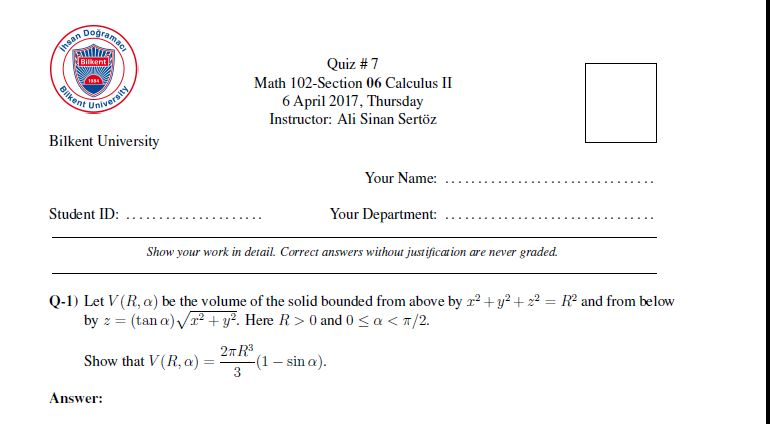For questions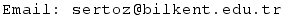Previous Screenshot       Next Screenshot